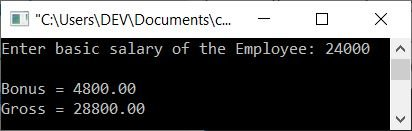# C Program for Calculating and Printing Bonus and Gross Salary Based on Basic Salary

In this article, we will learn how to create a program in C that will ask the user to enter his or her basic salary as input and then compute the bonus amount (bonus will be calculated at 20% of the basic salary) and gross salary using the given basic salary of the employee.

```#include<stdio.h>
#include<conio.h>
int main()
{
float basic, bonus, gross;
printf("Enter basic salary of the Employee: ");
scanf("%f", &basic);
bonus = (basic*20)/100;
gross = bonus + basic;
printf("\nBonus = %0.2f", bonus);
printf("\nGross = %0.2f", gross);
getch();
return 0;
}```

As the above program was written in the Code::Blocks IDE, here is the first snapshot of the sample run:Provide the basic salary of the employee, and the program will print the Bonus@20% and gross salary of the employee as shown here in the second snapshot of the sample run:Here are some of the main steps used in the above program:

• Receive the basic salary of the employee as input.
• Now calculate the bonus amount by applying 20 percent of the basic salary.
• And then calculate the gross salary by adding the bonus and the basic salary amount.
• Print the value of both the bonus and the gross salary amount as output.
• Here we have used the format specifier %0.2f to print only two digits after the decimal.

Before closing the discussion on this post, I'm willing to include one more example that does the same job of calculating and printing the bonus and gross salary based on the basic one. I used comments to describe the main codes used in the following C program:

C Code
```#include <stdio.h>

int main() {
float basic_salary, bonus, gross_salary;

printf("Enter basic salary: ");
scanf("%f", &basic_salary);

// Calculate bonus based on basic salary
if (basic_salary <= 10000) {
bonus = basic_salary * 0.1;
} else if (basic_salary <= 20000) {
bonus = basic_salary * 0.15;
} else {
bonus = basic_salary * 0.2;
}

// Calculate gross salary
gross_salary = basic_salary + bonus;

// Print bonus and gross salary
printf("Bonus: %.2f\n", bonus);
printf("Gross salary: %.2f\n", gross_salary);

return 0;
}```
Output
```Enter basic salary: 25000
Bonus: 5000.00
Gross salary: 30000.00```

C Quiz

« Previous Program Next Program »